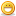# Thread: learn a bunch of languages real quick

1. ## learn a bunch of languages real quick

I thought this was a pretty damn cool idea, I figured some of you would have fun messing around with it as well.

http://www2.latech.edu/~acm/HelloWorld.shtmlReply With Quote

2. Code:
```#include "stdio.h"
#define e 3
#define g (e/e)
#define h ((g+e)/2)
#define f (e-g-h)
#define j (e*e-g)
#define k (j-h)
#define l(x) tab2[x]/h
#define m(n,a) ((n&(a))==(a))

long tab1[]={ 989L,5L,26L,0L,88319L,123L,0L,9367L };
int tab2[]={ 4,6,10,14,22,26,34,38,46,58,62,74,82,86 };

main(m1,s) char *s; {
int a,b,c,d,o[k],n=(int)s;
if(m1==1){ char b[2*j+f-g]; main(l(h+e)+h+e,b); printf(b); }
else switch(m1-=h){
case f:
a=(b=(c=(d=g)&lt;&lt;g)&lt;'&lt;g)&lt;&lt;g;
return(m(n,a|c)|m(n,b)|m(n,a|d)|m(n,c|d));
case h:
for(a=f;a=e)for(b=g&lt;&lt;g;b&lt;n;++b)o[b]=o[b-h]+o[b-g]+c;
return(o[b-g]%n+k-h);
default:
if(m1-=e) main(m1-g+e+h,s+g); else *(s+g)=f;
for(*s=a=f;a&lt;e; ) *s=(*s&lt;&lt;e)|main(h+a++,(char *)m1);
}
}```
WTF???

Heh, I can't make any sense of that code. Tried compiling it but didn't work. And I don't know how to fix the errorsBrain***** looked like a pretty interesting language. I tried to go to the web site but the link was broken or something.

Anyways, nice find PopinskymjkReply With Quote

3. It makes no sense to me either, but I think the code is incomplete (what's the purpose of tab1 and tab2 array?), or, I'm trying to run it on a wrong platform (Windowz).

Anyway I can get it compiled (Dev-C++ on XP) by removing the (ambiguous) single quote in line 19 and adding the (missing) semicolon in the first for in line 22. Still when executed it crashed with the usual friendly message:
"hello.exe has encountered a problem and needs to close. We are sorry the inconvenience."

Peace always,
&lt;jdenny&gt;Reply With Quote

####Posting Permissions

• You may not post new threads
• You may not post replies
• You may not post attachments
• You may not edit your posts
•